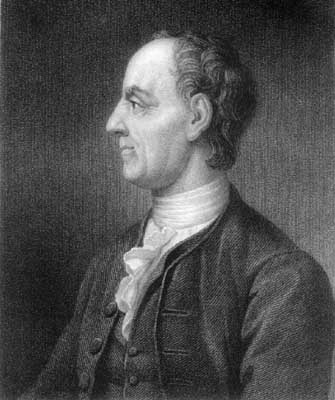# Leonhard Euler (1707-1783) mathematician

 ".. indeed, far and away the most prolific writer in the history of the subject" writes Howard Eves in An Introduction to the History of Mathematics. Euler's contribution to mathematics is represented here by a few of the notations conventionalized by him or in his honor. Around the world, these are read, written, and spoken thousands of times every day: e for the base of the natural logarithm (a.k.a. "the calculus number") a, b, c for the sidelengths of a triangle ABC f(x) for functional value R and r for the circumradius and inradius of a triangle sin x and cos x for values of the sine and cosine functions i for the imaginary unit, the "square root of -1" capital sigma for summation. capital delta for finite difference. Euler grew up near Basel, Switzerland, and studied at an early age under Johann Bernoulli. He finished studies at the University of Basel when only 15 years old. From 1727 to 1741, Euler worked in St. Perersburg, Russia, and then moved to the Akademie in Berlin. In 1766 he returned to St. Petersburg, where he remained.Two publications marking the bicentennial of Euler's death are of particular note:

Leonhard Euler 1707-1783, Beiträge zu Leben und Werk, Gedenkband des Kantons Basel-Stadt, edited by J. J. Burckhardt, E. A. Fellmann, and W. Habicht, Birkhäuser Verlag, Basel, 1983.

"A Tribute to Leohnard Euler 1707-1783, Mathematics Magazine, vol. 56, no. 5, November 1983. This issue includes seven articles:

J. J. Burckhardt, "Leonhard Euler, 1707-1783," 273-277.

G. L. Alexanderson, "Ars Expositionis: Euler as Writer and Teacher," 274-278.

George E. Andrews, "Euler's Pentagonal Number Theorem," 279-284.

Harold M. Edwards, "Euler and Quadratic Reciprocity," 285-291.

Paul Erdös and Underwood Dudley, "Some Remarks and Problems in Number Theory Related to the Work of Euler," 292-298.

Jesper Lützen, "Euler's Vision of a General Partial Differential Calculus for the Generalized Kind of Function," 299-306.

The articles are followed by a glossary of well known terms named for Euler, including:

Euler angles, Euler characteristic, Euler circle, Euler circuit, Euler-Mascheroni constant, Euler line (link below), Euler numbers, Eulerian numbers, Euler triangle, Euler's first integral (beta function), Euler's second integral (gamma function), Euler polynomials, Euler's phi function, Euler's identity (zeta function), Euler force (critical load), Euler's formula (e to the i theta - cos of theta + i sin of theta), Euler-Maclaurin summation formula, Euler's equation (in the calculus of variations), Euler's equation (in differential equations), Euler's equation of motion of an ideal fluid, Euler's equations for the rotation of a rigid body, Euler's equation on normal curvature, Euler multiplier, Euler method and improved Euler method for differential equations, Euler's addition theorem for elliptic integrals, Euler's criterion for quadratic residues, Fermat-Euler theorem, Euler-Lagrange Theorem (every positive integer is a sum of at most four squares), Euler's officer problem, Euler's theorems on partitions, Euler's theorem for four collinear points, Euler's theorem for polyhedra (V - E + F = 2), Euler's theorem for primes, Euler's theorem for rotation of a coordinate system, Eulers' theorem for a triangle (d^2 = R(R - 2r), where d is the distance between the circumcenter and incenter).

A rich source of information on Euler's work in number theory, mechanics, analysis, music theory, cartography, and naval science, including a long list of references, is

Ronald Calinger, "Leonhard Euler: The First St. Petersburg Years (1727-1741)," Historia Mathematica 23 (1996) 121-166.